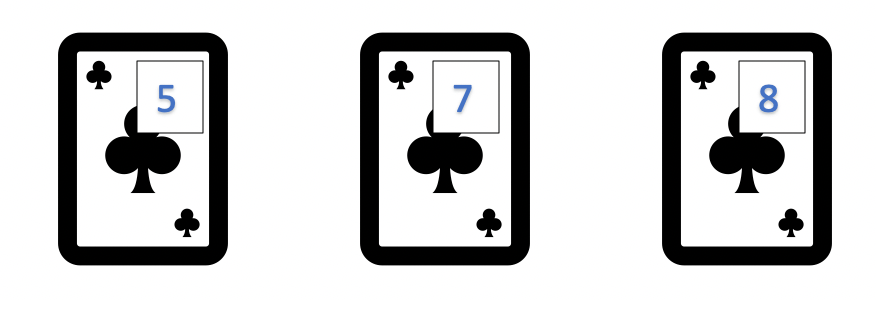# Olympiad Math Preparation – Class 2

## Number Sense – Comparing Numbers

1. 7 hundred forty five is ___________________ 7 hundred fifty

2. Which of below boxes has least number ?

3. Largest one digit number is _______________

4. Which of following number has largest value ?

5. Largest two digit number is _______________

6. Which of below balance is correct ?

7. Duggu has three cards. What is least 3 digit number that can be formed using each card only once

8. Which of below coin boxes have greatest number of coins ?

9. Largest three digit number is ________________

10. Which of following number is more greater than 450 and less than 575 in the screen below ?

11. The smallest 3 digit number is ___________